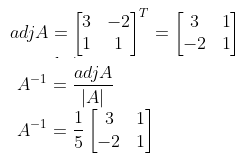# RBSE Maths Class 12 Chapter 5: Inverse of a Matrix and Linear Equations Important Questions and Solutions

RBSE Class 12 Maths Chapter 5 – Inverse of a Matrix and Linear Equations Important questions and solutions are available here. All these important questions are given here and have detailed stepwise solutions. The RBSE Class 12 important questions and solutions given at BYJU’S are important from the examination point of view.

Chapter 5 of RBSE Class 12 has only two exercises which cover various concepts of matrices such as singular, non-singular matrix, adjoint of a given matrix. Besides, different types of questions given here, such as finding the inverse of a matrix, finding the area of a triangle using determinant for the given vertices, solving a given system of equations using various methods involving matrix namely Cramer’s rule.

### RBSE Maths Chapter 5: Exercise 5.1 Textbook Important Questions and Solutions

Question 1: For what value of x, is the matrix

$$\begin{array}{l}\begin{bmatrix} 1 & -2 & 3\\ 1 & 2 & 1\\ x & 2 & -3 \end{bmatrix}\end{array}$$
singular?

Solution:

We know that if a matrix is singular, then its determinant is 0.

Thus,

$$\begin{array}{l}\begin{vmatrix} 1 & -2 & 3\\ 1 & 2 & 1\\ x & 2 & -3 \end{vmatrix}=0\end{array}$$

⇒ 1 (-6 – 2) + 2 (-3 – x) + 3(2 – 2x) = 0

⇒ -8 – 6 – 2x + 6 – 6x = 0

⇒ -8x = 8

⇒ x = (-8/8) = -1

Therefore, the required value of x = -1.

Question 2: If matrix A is

$$\begin{array}{l}\begin{bmatrix} 1 & -1 & 2\\ 3 & 0 & -2\\ 1 & 0 & 3 \end{bmatrix}\end{array}$$

Solution:

Given matrix is:

$$\begin{array}{l}A = \begin{bmatrix} 1 & -1 & 2\\ 3 & 0 & -2\\ 1 & 0 & 3 \end{bmatrix}\end{array}$$

Cofactors of matrix A are: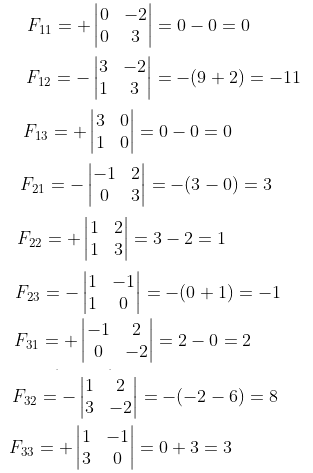Thus, the cofactor matrix of A

$$\begin{array}{l}=\begin{bmatrix} 0 & -11 & 0\\ 3 & 1 & -1\\ 2 & 8 & 3 \end{bmatrix}\end{array}$$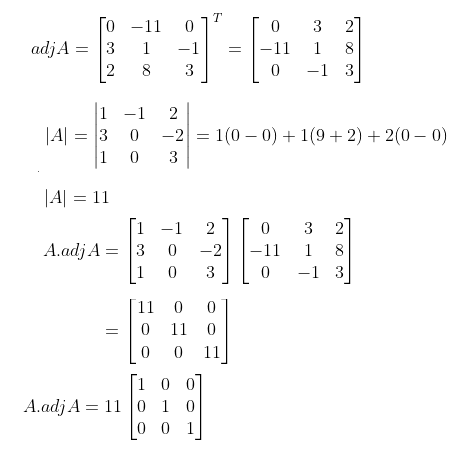A.(adj A) = |A| I3 ….(i)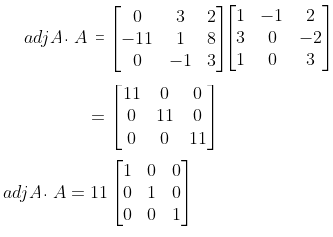(adj A).A = |A| I3 ….(ii)

From (i) and (ii),

Hence proved.

### RBSE Maths Chapter 5: Exercise 5.2 Textbook Important Questions and Solutions

Question 3: Find the area of the triangle using the determinants whose vertices are (0, 0), (5, 0) and (3, 4).

Solution:

Given,

Vertices of the triangle are (0, 0), (5, 0) and (3, 4).

Area of triangle

$$\begin{array}{l}= \frac{1}{2}\begin{vmatrix} 0 & 0 & 1\\ 5 & 0 & 1\\ 3 & 4 & 1 \end{vmatrix}\end{array}$$

= 1/2 [0(0 – 4) – 0(5 – 3) + 1(20 – 0)]

= 1/2(0 – 0 + 20)

= 20/2

= 10 sq.units

Question 4: Solve the following system of equations using Cramer’s rule.

2x + 3y = 9

3x – 2y = 7

Solution:

Given system of equations are:

2x + 3y = 9

3x – 2y = 7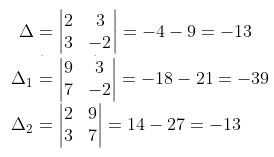Δ ≠ 0, Δ1 ≠ 0, Δ2 ≠ 0

Thus, the solution will be unique.

x = Δ1/Δ = (-39)/(-13) = 3

y = Δ2/Δ = (-13)/(-13) = 1

Therefore, the solution of the given system of equations is x = 3 and y = 1.

### RBSE Maths Chapter 5: Additional Important Questions and Solutions

Question 1: If

$$\begin{array}{l}A=\begin{bmatrix} 1 & -1\\ 2 & 3 \end{bmatrix}\end{array}$$
, then find A-1.

Solution:

Given,

$$\begin{array}{l}A=\begin{bmatrix} 1 & -1\\ 2 & 3 \end{bmatrix}\end{array}$$

|A| = 3 – (-2) = 3 + 2 = 5

|A| ≠ 0

Thus, A-1 exists.

F11 = 3, F12 = -2, F21 = 1, F22 = 1

Cofactor matrix of A

$$\begin{array}{l}=\begin{bmatrix} 3 & -2\\ 1 & 1 \end{bmatrix}\end{array}$$